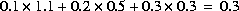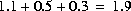[Next] [Previous] [Up] [Top]

Keywords

### MIX

This keyword data block is used if two or more aqueous solutions are to be mixed together. The mixing occurs as part of the reaction calculation.

#### Example

```Line 0:  MIX 2 Mixing solutions 5, 6, and 7.
Line 1a:      5     1.1
Line 1b:      6     0.5
Line 1c:      7     0.3
```

#### Explanation

Line 0: MIX [number] [description]

MIX is the keyword for the data block.

number--positive number to designate these mixing parameters. Default is 1.

description--optional character field that describes the mixture.

Line 1: solution number, mixing fraction

solution number--defines a solution to be part of the mixture.

mixing fraction--positive, decimal number which is multiplied times the concentrations of each element in the specified solution. Mixing fractions may be greater than 1.0.

#### Notes

In mixing, each solution is multiplied by its mixing fraction and a new solution is calculated by summing over all of the fractional solutions. In the example, if the number of moles of sodium in solutions 5, 6, and 7 were 0.1, 0.2, and 0.3, the number of moles of sodium in the mixture would be. The moles of all elements are multiplied by the solution's mixing fraction, including hydrogen and oxygen. Thus, the mass of water is effectively multiplied by the same fraction. In the example, if all solutions have 1 kg of water, the total mass of water in the mixture iskg and the concentration of sodium would be approximately 0.16 molal. The charge imbalance of each solution is multiplied by the mixing fraction and all the imbalances are then summed to calculate the charge imbalance of the mixture. The temperature of the mixture is approximated by multiplying each solution temperature by its mixing fraction, summing these numbers, and dividing by the sum of the mixing fractions. Other intensive properties of the mixture are calculated in the same way as temperature.

This formulation of mixing can be used to approximate constant volume processes if the sum of the mixing fractions is 1.0 and all of the solutions have the same mass of water. The calculations are only approximate in terms of mixing volumes because the summation is actually made in terms of moles (or mass) and the volumes of solutions are not known. Similarly, the formulation for mixing can approximate processes with varying volume, for example, a titration.

#### Example problems

The keyword MIX is used in example problems 3 and 4.

#### Related keywords

SOLUTION, SAVE solution, USE solution, and USE mix.

Example
Explanation
Notes
Example problems
Related keywords

User's Guide to PHREEQC - 07 MAY 96
[Next] [Previous] [Up] [Top]